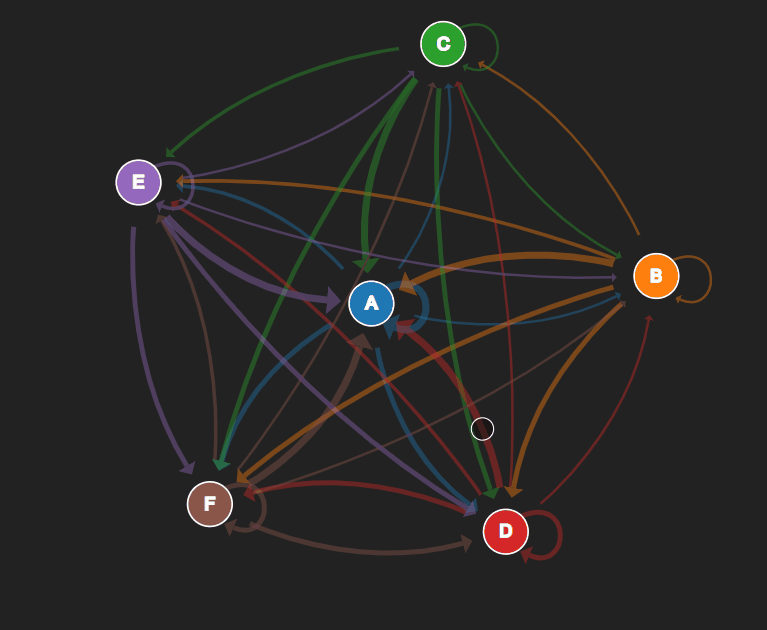Photo by Jason Leung on Unsplash

# Creating a Markov Transition Matrix from a streaming data source

## Using a “markovian” streaming data source to create a real-time Markov transition matrix

With this method, many useful insights can arise: such as comparing the matrices of different sources to see if there are statistically significant differences in probabilities (where there should be none) or comparing signals in time (weekdays vs weekends, or differences by hour).

You will likely notice that there are few naturally occurring stochastic processes that can be classified as a strong markov process. However, many naturally occurring processes can have a weak dependence on previous states, so much so that they can be analyzed as a traditional markov process.

One example is the market movements of a single security in terms of the directional movement from state to state, e.g. UP, SAME, DOWN.

Another example is the state of a customer within an established sequential framework, e.g from UNAWARE, NON-INTERESTED, INTERESTED, PURCHASER. Although the transitions between states are not as discrete, analyzing the state changes over longer periods of time can lead to interesting insights, such as actions that affect the emission probabilities between interested and purchaser over subsets of your customer population.

# Primer on Markov processesa 2 state memoryless Markov process. source: https://setosa.io/

A brief primer on Markov processes —

A discrete time Markov process is one in which the next state, denoted by `X_n+1`, depends only on the current state, X_n. Formally defined as:

Pretty simple.

Let’s break this into a simple example. We have an imaginary world with simplified weather, it’s either sunny or rainy. The probability of rain tomorrow (`X_n+1`) when it’s sunny today (X_n) is 0.6, the probability of sun when it’s rainy is 0.8. This gives us the following transition matrix. Note here that the probabilities of the rows add to 1. Each entry in the transition matrix is just the conditional probability distribution of the dependent states

`[[Pr(sun|sun) = 0.4, Pr(rain|sun) = 0.6],[Pr(sun|rain) = 0.8, Pr(rain|rain) = 0.2]]`

Visualized, this looks something like this:

# Creating a Markov transition matrix from a streaming data source

So, now that we understand the math, we can build a simple class to “observe” data coming in from our Markov process. This class constructs our Markov transition matrix from a series of unique states (ints or strings), then as each new data is observed, holds a count of the state observations, and on demand, constructs a real time view of our emission probabilities.

See the code below. In this example, I create a set of 6 distinct states [p, q, r, x, y, z]. It is assumed that each of these is observed in a sequence. From there, I generate observations according to a predefined probability distribution. Then, observe each with my MarkovTransitionMatrix class.

With this class, you can easily set up a new streaming data source, such as a scikit-multiflow data source and use this to keep a running Markov transition matrix.An example of the 6 state markov process.

There are a number of interesting things to do with MTMs from various streams. I’ve found interesting insights by calculating the KL Divergence of two related streams. Now — it’s up to you. Enjoy!

A special thanks to the folks at Setosa.io. If you liked these visualizations, check out: https://setosa.io/

If you liked this article, you might also like:

Thanks for reading!

Data Scientist